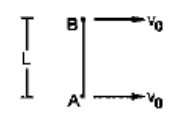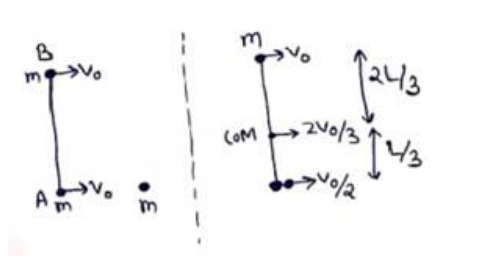# Solve the following :Question:

Two small balls $A$ and $B$, each of mass $m$, are joined rigidly by a light horizontal rod of length $L$. The system translates on a frictionless horizontal surface with a velocity $v_{0}$ in a direction perpendicular to the rod. A particle $P$ of mass $m$ kept at rest on the surface sticks to the ball $A$ as the ball collides with it. Find

(a) the linear speeds of the balls $A$ and B after the collision, (b) the velocity of the centre of mass $C$ of the system $A+B+P$ and (c) the system about $C$ after the collision.[Hint: The light rod will exert a force on the ball B only along its length.]

Solution:

(a) The light rod will exert a force on the ball B only along its length. So collision will not effect its velocity.

$v_{B}=v_{O}$

Momentum conservation for ball $A$

$m^{v_{O}}=2 m^{v_{A}}$

$v_{A}=\frac{v_{0}}{2}$

(b)$\overrightarrow{v_{C M}}=\frac{m_{1} \overrightarrow{v_{1}}+m_{2} \overrightarrow{v_{2}}}{m_{1}+m_{2}}$

$=\frac{m w 0+2 m\left(\frac{\mathrm{mO}}{\mathrm{n}}\right)}{m+2 m}$

$\overrightarrow{v_{C M}}=\frac{2 v_{0}}{3}$